## Test Prep Plan - Take a practice test

Take this practice test to check your existing knowledge of the course material. We'll review your answers and create a Test Prep Plan for you based on your results.
How Test Prep Plans work
1
2Based on your results, we'll create a customized Test Prep Plan just for you!
3Study smarter
Study more effectively: skip concepts you already know and focus on what you still need to learn.

# Using Derivatives Chapter Exam

Exam Instructions:

Choose your answers to the questions and click 'Next' to see the next set of questions. You can skip questions if you would like and come back to them later with the "Go To First Skipped Question" button. When you have completed the practice exam, a green submit button will appear. Click it to see your results. Good luck!

### Page 1

#### Question 1 1. Which of the following BEST describes point C?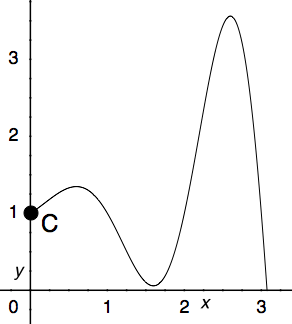#### Question 4 4. Where is the global maximum for the function y=f(x) below between x=0 and x=2?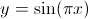### Page 2

#### Question 6 6. Which of the following is a local minimum?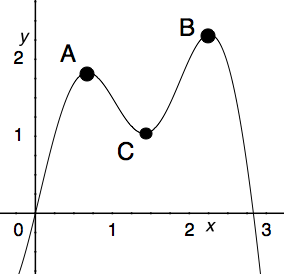#### Question 7 7. What is the formula for your bank balance given the following conditions: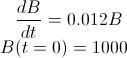#### Question 8 8. If h equals 3 m, and x is moving at a rate of 3 m / second, how fast is L changing when x equals 4m?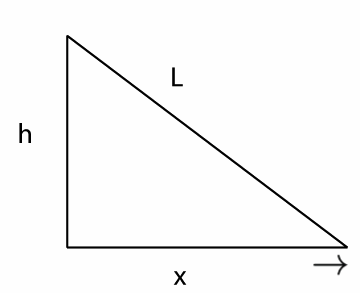#### Question 9 9. What is the global minimum for the function y=f(x) below between x=0 and x=2?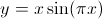#### Question 10 10. Solve the following differential equation for c=f(a).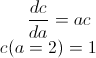### Page 3

#### Question 11 11. Which of the following BEST describes point B?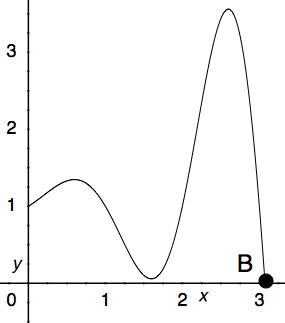#### Question 12 12. In the following right triangle, what is the equation for L as a function of x?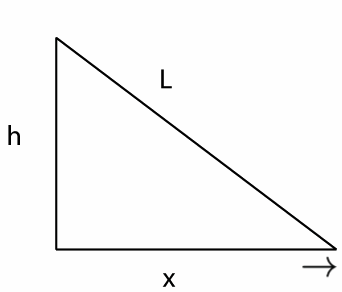### Page 4

#### Question 16 16. You have a tank that is shaped like a prism that is on its side. The triangular base of the tank is a right triangle of height 10 m and width 10 m. See the image below. If the tank is being filled at a rate of 30 cubic meters per minute, how quickly is the height changing?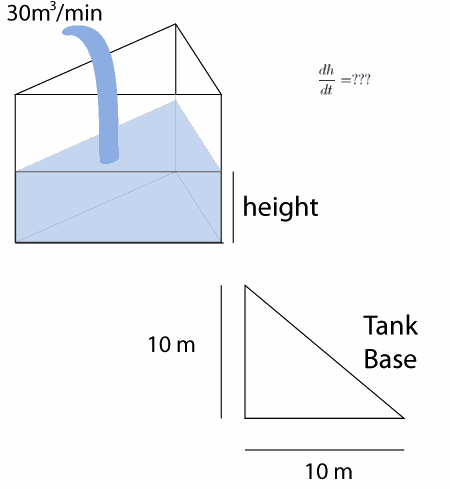#### Question 17 17. Solve the following differential equation for y=f(x).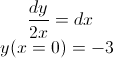#### Question 18 18. The red car is traveling at 45 mph and the blue car is traveling toward the red car but is braking so that v(t) = 60 - 30t. In how many hours will they pass each other, assuming that they are 10 miles apart at t = 0?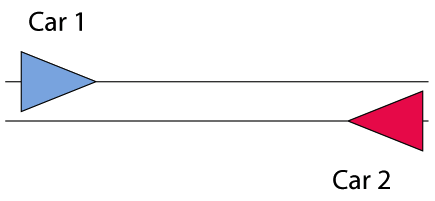#### Question 19 19. Which of the following BEST describes point A?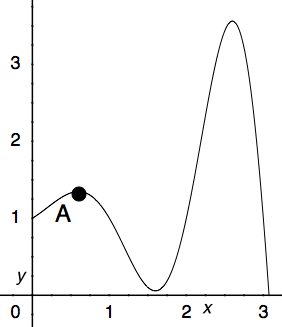### Page 5

#### Question 21 21. Solve the following differential equation for y=f(x) (assume that y is always positive).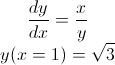#### Question 23 23. What is the global minimum for the function y=f(x) below between x=0 and x=2?#### Question 24 24. What is value of y at the finite local maximum for the function y=f(x) below?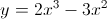### Page 6

#### Question 28 28. The following differential equation represents the change in population as a function of time. What does the 0.03 represent in this equation?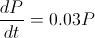#### Question 29 29. What is the equation of how L changes as a function of how x changes if h=6?#### Question 30 30. The red and blue cars are 10 miles apart, but headed toward one another. The red car is traveling at 45 mph, and the blue car is traveling at a speed v(t). What is the equation for how the distance between them changes as a function of time?#### Using Derivatives Chapter Exam Instructions

Choose your answers to the questions and click 'Next' to see the next set of questions. You can skip questions if you would like and come back to them later with the "Go To First Skipped Question" button. When you have completed the practice exam, a green submit button will appear. Click it to see your results. Good luck!

Support